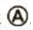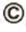Chapter 5, Problem 63AP

Chapter
Section
Textbook Problem

A roller-coaster car of mass 1.50 × 103 kg is initially at the top of a rise at point. It then moves 35.0 m at an angle of 50.0° below the horizontal to a lower point. (a) Find both the potential energy of the system when the car is at pointsandand the change in potential energy as the car moves from pointto point, assuming y = 0 at point. (b) Repeat part (a), this time choosing y = 0 at point, which is another 15.0 m down the same slope from point.

(a)

To determine
Bothe the potential energy of the system when the car is on A and B and the change in potential energy of the car when it moves from A to B, when y=0 at point B.

Explanation

Section1:

To determine: The potential energy of the system at point A.

Answer: the potential energy of the system at point A is 3.94×105J .

Explanation:

Given Info:

The mass of the roller-coaster car is 1.50×103kg .

The distance that the car travelled between point A and B is 35.0m .

The path is at an angle 50.0° below the horizontal to the lower point B.

Since y=0 is considered at point B,

yB=0

yA=lsinθ       (I)

• yB is the height to the point B from the point y=0
• yA is the height to the point A from the point y=0
• l is the length that the car moves
• θ is the angle of the path of the car to the horizontal

Formula to calculate the potential energy of the system at point A is,

PEA=mgyA (II)

• m is mass of the car
• g is acceleration due to gravity

Substitute equation (I) in (II),

PEA=mg(lsinθ)

Substitute 1.50×103kg for m , 9.8m/s2 for g, 35.0m for l and 50.0° for θ to find the potential energy of the system at point A,

PEA=(1.50×103kg)(9

(b)

To determine
Bothe the potential energy of the system when the car is on A and B and the change in potential energy of the car when it moves from A to B, when y=0 at point C which is 15.0m down the slope from point B.

Still sussing out bartleby?

Check out a sample textbook solution.

See a sample solution

The Solution to Your Study Problems

Bartleby provides explanations to thousands of textbook problems written by our experts, many with advanced degrees!

Get Started

Find more solutions based on key concepts